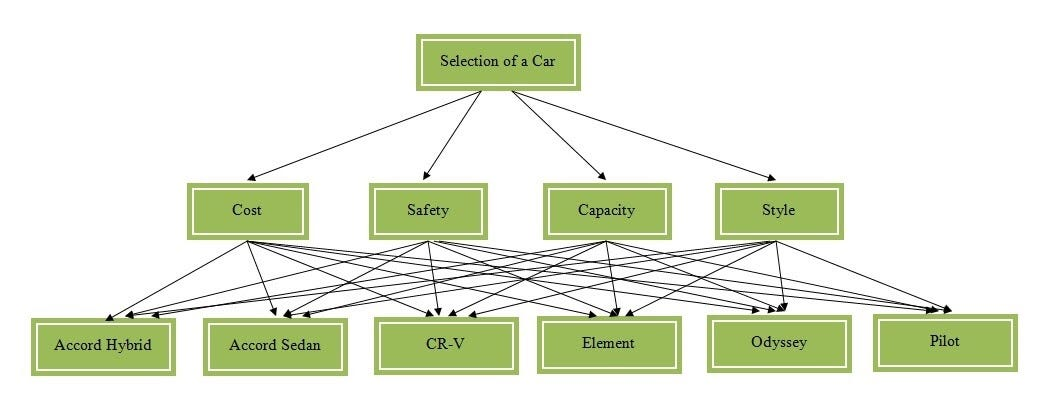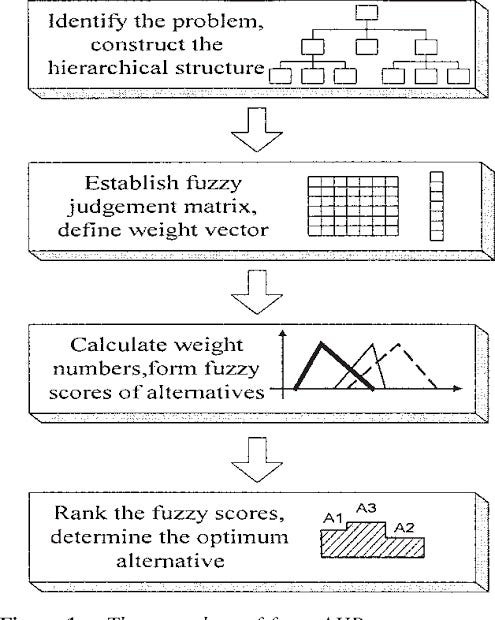# A Fuzzy Analytical Hierarchy Process (FAHP) using R

## What is Fuzzy Analytical hierarchical process

The manager’s ability to produce optimal decisions are critical for any company to remain competitive. Fuzzy analytical hierarchical process could be a very useful tool to facilitate decision making of managers. Fuzzy logic is a very useful tool to transform qualitative assessments of a human to quantitative assessment by taking uncertainty into account. In a simple Analytical hierarchy process model, a decision maker has to do a weight scaling by carrying out comparisons in pair for his criteria and alternatives. He has to enter a definite number for his preferences, but in real world situation, a decision maker is not sure about the numerical values (weights) he should assign for his preferences. Fuzzy Analytical hierarchical process takes the real world uncertainty into account.

In fuzzy analytical hierarchical process, triangular membership functions are used instead of a single definite number. Therefore, scaling for FAHP is done according to the following table.

# Implementation of fuzzy analytics hierarchy process for selecting a car model based on certain criteria using R.

Let us consider an example. Suppose an engineer Siddhartha wants to purchase a car and he has chosen six car models for evaluation. He has four criteria based on which he will select a car model to be purchased. The criteria for his selection are cost, safety, capacity, and style. The four car models (Alternatives) to chose from are Accord-hybrid, Accord-sedan, CR-V, Element, Odyssey, and Pilot.

The hierarchical structure of the car problem is shown in figure-1.Figure-2: The hierarchical structure of the car model selection problem

The FAHP process flow is shown in Figure- 2.Figure-3: Fuzzy Analytical process flow diagram

The fuzzy weight matrix for criteria is shown below

In my R implementation, I have simplified the entry of weights for criteria and alternatives.

Say for example criteria weight matrix i.e. an array C of dimension n by n by 3 is created as below. here n=4 as there are for criteria.

`C <- array(NA,dim = c(n,n,3))`

C[i,j,1:3] represents the fuzzy weights for criteria i w.r.t j. for example C[1,3, ] = c(6,7,8) means criteria 1 is very strongly more important than criteria 3. Four entries C[1,1,] to C[1,4,] is entered manually. The function MatrixFiller(A) fills the rest entry of the matrix based on the weights provided by the user for the first four entry.

`C[1,1,] = c(1,1,1)C[1,2,] = c(8,8,8)C[1,3,] = c(6,7,8)C[1,4,] = c(1,2,3)C <- MatrixFiller(C)`

The code for MatrixFiller function is as below.

`MatrixFiller <- function(A){  s2 <- dim(A)  for(i in 2:s2)  {    for(j in 1:s2)    {      A[i,j,] <- A[1,i,]/A[1,j,]    }  }  return(A)}`

similarly, the weight matrix for alternatives are prepared. the dimension for matrix for Alternatives would be of 6 by 6 by 3.

The entire R programming code for Car model selection problem is given below

`#Enter criteria # Enter the number of Criteria# As in the case of Carexample# the number of criteria is 4 that are 1. Cost 2. Safety 3. Capacity 4. Style# n is the number of Criterialibrary(psych)library(ggplot2)n <- 4# The criteria weight matrix C <- array(NA,dim = c(n,n,3))#Assignment of pair-wise weight for criteria C[1,2,] means prefrence of#Criteria 1 over Criteria 2#Calculation of MAtrixA <- A1MatrixFiller <- function(A){  s2 <- dim(A)  for(i in 2:s2)  {    for(j in 1:s2)    {      A[i,j,] <- A[1,i,]/A[1,j,]    }  }  return(A)}C[1,1,] = c(1,1,1)C[1,2,] = c(8,8,8)C[1,3,] = c(6,7,8)C[1,4,] = c(1,2,3)C <- MatrixFiller(C)new.function <- function(P){  s <- dim(P)  n <- s  D <- matrix(NA, nrow = n, ncol = 3)  for(i in 1:3)  {    for(j in 1:n)    {      D[j,i] <- geometric.mean(c(P[j,1,i],P[j,2,i],P[j,3,i],P[j,4,i]))    }  }  return(D)}#Geometric mean of fuzzy comparison valueD1 <- new.function(C)Cw <- rowMeans(D1)/sum(rowMeans(D1))# Calculation for weights for Alternatives# Entering pair-wise weights for Criteria 1: Cost for Alternatives# In Car example Alternatives are A1: Accord Sedan, A2: Accord Hybrid# A3: Pilot, A4: CR-V, A5: Element, A6: Odyssey# Entering weights w.r.t criteria CostN <- 6A1 <- array(NA,dim = c(N,N,3))A1[1,1,] = c(1,1,1)A1[1,2,] = c(2,3,4)A1[1,3,] = c(5,6,7)A1[1,4,] = c(1,2,3)A1[1,5,] = c(3,4,5)A1[1,6,] = c(6,7,8)A1 <-MatrixFiller(A1)D2 <- new.function(A)A1w <- rowMeans(D2)/sum(rowMeans(D2))#Entering weights w.r.t Criteria safetyN <- 6A2 <- array(NA,dim = c(N,N,3))A2[1,1,] = c(1,1,1)A2[1,2,] = c(6,7,8)A2[1,3,] = c(1,2,3)A2[1,4,] = c(1,2,3)A2[1,5,] = c(9,9,9)A2[1,6,] = c(6,7,8)A2 <- MatrixFiller(A2)D3 <- new.function(A2)A2w <- rowMeans(D3)/sum(rowMeans(D3))#Entering Weights for Criteria w.r.t CapacityN <- 6A3 <- array(NA,dim = c(N,N,3))A3[1,1,] = c(1,1,1)A3[1,2,] = c(1,2,3)A3[1,3,] = c(9,9,9)A3[1,4,] = c(1,2,3)A3[1,5,] = c(1,2,3)A3[1,6,] = c(6,7,8)A3 <- MatrixFiller(A3)D4 <- new.function(A3)A3w <- rowMeans(D4)/sum(rowMeans(D4))#Entering Criteria w.r.t. StyleN <- 6A4 <- array(NA,dim = c(N,N,3))A4[1,1,] = c(1,1,1)A4[1,2,] = c(9,9,9)A4[1,3,] = c(4,5,6)A4[1,4,] = c(1,2,3)A4[1,5,] = c(3,4,5)A4[1,6,] = c(6,7,8)A4 <- MatrixFiller(A4)D5 <- new.function(A4)A4w <- rowMeans(D5)/sum(rowMeans(D5))Alt_MAt <- matrix(c(A1w,A2w,A3w,A4w), nrow = N)Crt_MAT <- matrix(Cw, nrow = 4)Final_Score <- Alt_MAt %*% Crt_MAT#create plotdata <- data.frame(CarModel = c("Accord Sedan", "Accord Hybrid","Pilot","CR-V","Element", "Odessey"), score = Final_Score)ggplot(data, aes(x=CarModel, y= score)) +   geom_bar(stat = "identity")`

Output is the final score calculated which is shown in the Figure-5